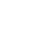• 支持90多种语言
我们可以将任何类型的文件翻译成 90 多种语言。
• 最优价格保证
我们能与网上高评价的竞争对手比价。
• 可选当日交稿
当您选择我们的标准头等速度时，大多数的文件将在 1-2 个工作日内送达。
为保证能当日交稿，请在下订单时选择加急翻译服务# 保证认可

1. 将文件从......语言翻译 :

• 中文(繁体)
• 英语
• 法语
• 西班牙语
• 中文(简体)
• 意大利语
• 葡萄牙语（欧洲）
• 葡萄牙语（巴西）
• 韩语
• 日语
• 阿拉伯语
• 俄罗斯语
• 德语
• 中文(简体)
• 中文(繁体)
• 丹麦语
• 乌克兰语
• 乌兹别克语
• 乌尔都语
• 亚美尼亚语
• 他加禄语（菲律宾）
• 俄罗斯语
• 保加利亚语
• 信德语
• 僧伽罗语
• 克伦语支
• 克罗地亚语
• 冰岛语
• 加利西亚语
• 加泰罗尼亚语
• 匈牙利语
• 南非语
• 卡纳达语
• 卢森堡语
• 印地语
• 印尼语
• 印尼语
• 古吉拉特语
• 吉尔吉斯语
• 哈萨克语
• 土耳其语
• 塔吉克语
• 塞尔维亚语
• 塞索托语
• 奇契瓦语
• 契维语
• 奥斯曼土耳其语
• 威尔士语
• 孟加拉语
• 宿务语
• 尼泊尔语
• 巴斯克语
• 巽他语
• 希伯来语
• 希腊语
• 广东话
• 库尔德语（Kurmanji）
• 弗拉芒语
• 弗里西亚语
• 德语
• 意大利语
• 意第绪语
• 拉丁语
• 拉脱维亚语
• 挪威语
• 捷克语
• 提格利亚语
• 斯洛伐克语
• 斯洛文尼亚文
• 斯瓦希里语
• 旁遮普语
• 日语
• 普什图语
• 柬埔寨语（高棉语）
• 格鲁吉亚语
• 毛利语
• 法语
• 波兰语
• 波斯尼亚语
• 波斯语
• 波斯语（波斯语）
• 泰卢固语
• 泰米尔语
• 泰语
• 海地克里奥尔语
• 爪哇语
• 爱沙尼亚语
• 瑞典语
• 白俄罗斯语
• 祖鲁族
• 科萨语
• 科西嘉语
• 立陶宛语
• 索马里语
• 约鲁巴语
• 绍纳语
• 缅甸语
• 罗马尼亚语
• 老挝语
• 芬兰语
• 苏格兰盖尔语
• 苗语
• 英语
• 荷兰语
• 菲律宾语（他加禄语）
• 萨摩亚语
• 葡萄牙语（巴西）
• 葡萄牙语（欧洲）
• 蒙古语
• 西印度克里奥尔语
• 西班牙语
• 豪萨语
• 越南语
• 达里语
• 阿塞拜疆语
• 阿姆哈拉语
• 阿尔巴尼亚语
• 阿拉伯语
• 韩语
• 马其顿语
• 马尔加什语
• 马拉塔语
• 马拉雅拉姆语
• 马来语
• 马耳他语
• 高棉语（柬埔寨语）
• 黑山语
2. 将文件翻译成 :

• 英语
• 中文(繁体)
• 法语
• 西班牙语
• 中文(简体)
• 意大利语
• 葡萄牙语（欧洲）
• 葡萄牙语（巴西）
• 韩语
• 日语
• 阿拉伯语
• 俄罗斯语
• 德语
• 中文(简体)
• 中文(繁体)
• 丹麦语
• 乌克兰语
• 乌兹别克语
• 乌尔都语
• 亚美尼亚语
• 他加禄语（菲律宾）
• 俄罗斯语
• 保加利亚语
• 信德语
• 僧伽罗语
• 克伦语支
• 克罗地亚语
• 冰岛语
• 加利西亚语
• 加泰罗尼亚语
• 匈牙利语
• 南非语
• 卡纳达语
• 卢森堡语
• 印地语
• 印尼语
• 印尼语
• 古吉拉特语
• 吉尔吉斯语
• 哈萨克语
• 土耳其语
• 塔吉克语
• 塞尔维亚语
• 塞索托语
• 奇契瓦语
• 契维语
• 奥斯曼土耳其语
• 威尔士语
• 孟加拉语
• 宿务语
• 尼泊尔语
• 巴斯克语
• 巽他语
• 希伯来语
• 希腊语
• 广东话
• 库尔德语（Kurmanji）
• 弗拉芒语
• 弗里西亚语
• 德语
• 意大利语
• 意第绪语
• 拉丁语
• 拉脱维亚语
• 挪威语
• 捷克语
• 提格利亚语
• 斯洛伐克语
• 斯洛文尼亚文
• 斯瓦希里语
• 旁遮普语
• 日语
• 普什图语
• 柬埔寨语（高棉语）
• 格鲁吉亚语
• 毛利语
• 法语
• 波兰语
• 波斯尼亚语
• 波斯语
• 波斯语（波斯语）
• 泰卢固语
• 泰米尔语
• 泰语
• 海地克里奥尔语
• 爪哇语
• 爱沙尼亚语
• 瑞典语
• 白俄罗斯语
• 祖鲁族
• 科萨语
• 科西嘉语
• 立陶宛语
• 索马里语
• 约鲁巴语
• 绍纳语
• 缅甸语
• 罗马尼亚语
• 老挝语
• 芬兰语
• 苏格兰盖尔语
• 苗语
• 英语
• 荷兰语
• 菲律宾语（他加禄语）
• 萨摩亚语
• 葡萄牙语（巴西）
• 葡萄牙语（欧洲）
• 蒙古语
• 西印度克里奥尔语
• 西班牙语
• 豪萨语
• 越南语
• 达里语
• 阿塞拜疆语
• 阿姆哈拉语
• 阿尔巴尼亚语
• 阿拉伯语
• 韩语
• 马其顿语
• 马尔加什语
• 马拉塔语
• 马拉雅拉姆语
• 马来语
• 马耳他语
• 高棉语（柬埔寨语）
• 黑山语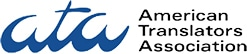• 英语
• 中文
• 葡萄牙语
• 意大利语
• 法语

•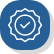支持90多种语言 我们可以将任何类型的文件翻译成 90 多种语言。
•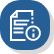质量保证 西班牙集团是美国翻译协会的成员，可以为所有翻译的质量做保证。我们对文件进行的审核不另收费，并且致力于提供五星级的体验。
•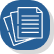可选当日交稿 当您选择我们的标准头等速度时，大多数的文件将在 1-2 个工作日内送达。为保证能当日交稿，请在下订单时选择加急翻译服务。
•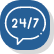安全付款 我们使用最先进的加密技术，让您所有的数据和信息都安全受保护。

### 我们热爱翻译

• 商业文件
• 民事证件（出生证、结婚证等)
• 员工手册
• 法律文件
• 网站
• 医疗文件
• 文学作品等

### 专业翻译# 我们精通90多种语言

• 保证认证翻译的接受度
• 无可比拟的准确度和高效性
• 绝对的文件保密性
• 商业、学术、法律等专业领域的中文译者

## 我们的翻译流程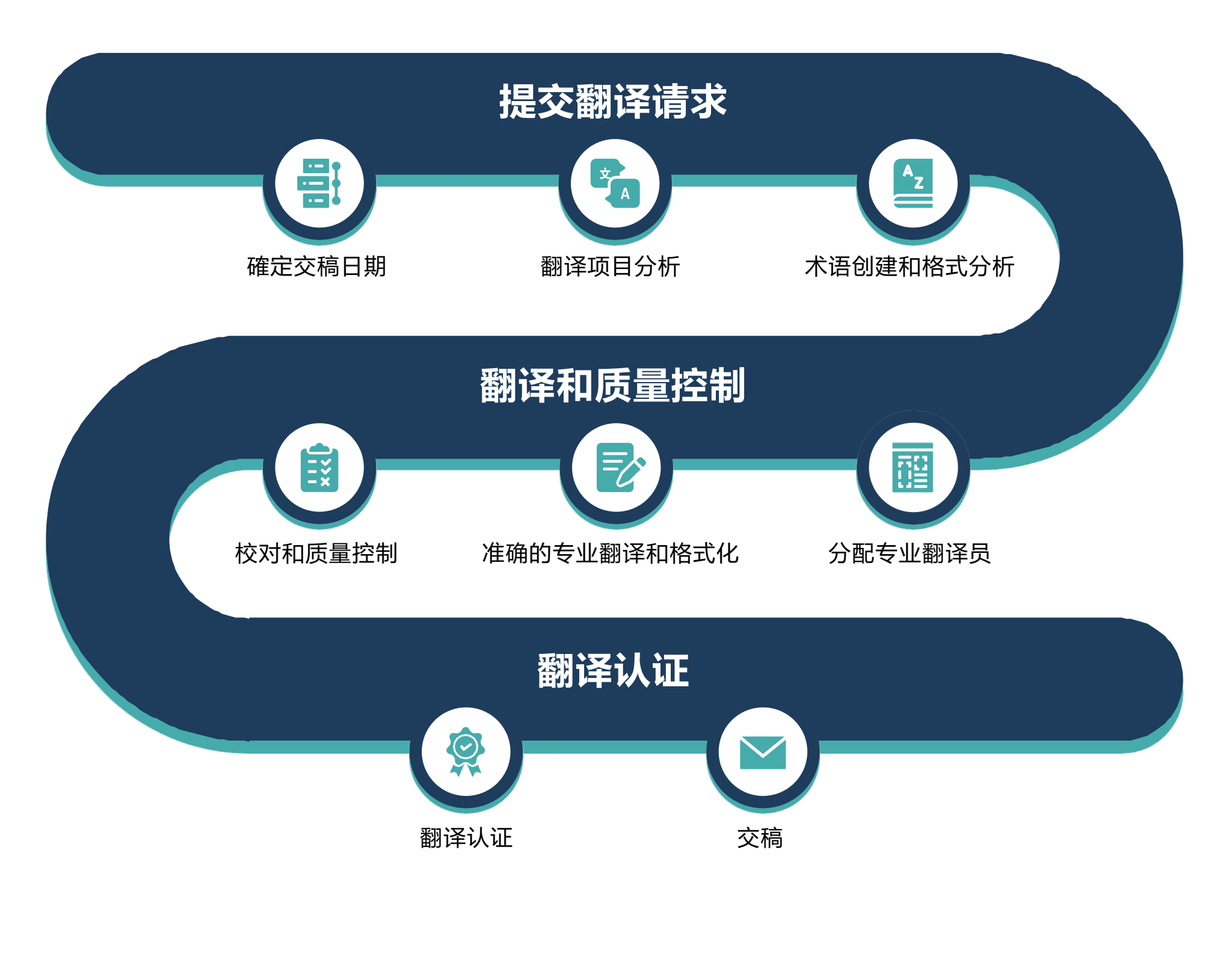The Spanish Group聘请了当今行业约1%的顶尖翻译人才。除了我们聘请的大量人才外，大多数情况下，The Spanish Group还可以确保您在不到48小时内获得准确认证翻译。您不需再百度搜索“我附近的翻译服务”，或在手机通讯录中寻找翻译，我们在此随时为您服务。

The Spanish Group专门为您提供满足您需求的特定语言翻译。只需告知我们您需要某一特定的方言专家，比如闽南话翻译等等，我们会乐意立即为您提供服务。

### 有问题吗？

+1 (800) 460-1536

info@thespanishgroup.org